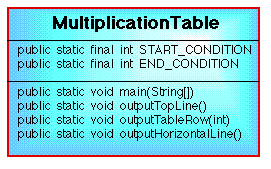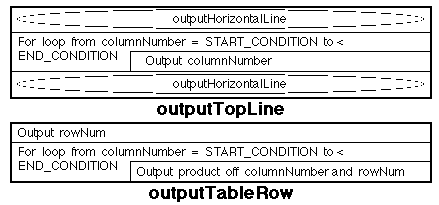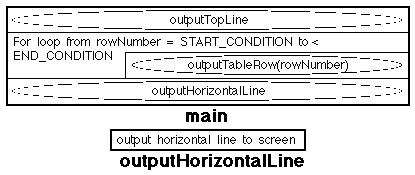# NOTE: This set of www pages is not the set of www pages for the curent version of COMP101. The pages are from a previous version that, at the request of students, I have kept on line.

## CONTENTS

 1. Overview 2. Example problem - Multiplication table 2.1. Requirements 2.2. Analysis ` ` 2.3. Design 2.4. Implementation 2.5. Testing 3. Alternative implementation

The random number generator example problem uses all three loop constructs in a variety of variable and fixed count modes.

## 1. OVERVIEW

As with selection statements loops can be nested. Care should be taken when nesting loops as this tends to decreases the readability of the code. Unless we are undertaking some fairly trivial nesting it is normally better to encode the nested loops in the form of further methods called from within the "parent" loop.

## 3. ALTERNATIVE IMPLEMENTATION (WITHOUT NESTED LOOPS)

 An alternative implementation might comprise four distinct methods: main, outputTopLine, outputTableRow and outputHorizontalLine. The advantage is that we avoid the loop nesting and make the main method less cluttered, therefore more readable and thus more maintainable. A class diagram for this proposed alternative implementation is presented in Figure 3. ` `Figure 3: Class diagram for multiplication table program (Version 2)
 Field Summary private static final int START_CONDITION            A ptrivate class constant, set to 1, marking the start value for the loop counter. private static final int END_CONDITION            A ptrivate class constant, set to 13, marking the end value for the loop counter.

 Method Summary public static void main(String[] args)            Main method. Calls outputTopLine method, then loops through row number calling outputTableRow method on each iteration. On completion of loop calls outputHorizontalLine method. private static void outputTopLine()            Outputs start of table: horizontal line, list of column numbers (using a loop construct) and another horizontal line. private static void outputTableRow(int rowNum)            Outputs elements in row of table using a loop construct. On each iteration the product of the current row and column numbers is output. private static void outputHorizontalLine()            Trivial method to output a line of '-' (hyphen) characters.

Appropriate Nassi-Shneiderman charts for the first three of the above methods are presented in Figure 4.Figure 4: Nassi-Shneiderman charts for multiplication table generator (Version 2)

The implementation is as given in Table 2.

 ```// MULTIPLICATION TABLE VERSION 2 // Frans Coenen // Thursday 17 May 1999 // The University of Liverpool, UK class MultiplicationTableVer2 { // ------------------- FIELDS ------------------------ private static final int startCondition = 1; private static final int endCondition = 13; // ------------------ METHODDS ----------------------- /** Main method */ public static void main(String[] args) { int rowNumber; // Output top line of table outputTopLine(); // Output table entries for(rowNumber = startCondition; rowNumber < endCondition; rowNumber++) { outputTableRow(rowNumber); } // Horizontal line outputHorizontalLine(); System.out.println("\n"); } /* Method to output top line of table. */ private static void outputTopLine() { int columnNumber; // Horizontal line System.out.println("\n"); outputHorizontalLine(); // Output top line of "coordinates" System.out.print("\t| "); for(columnNumber = startCondition; columnNumber < endCondition; columnNumber++) { System.out.print(columnNumber + "\t "); } // Horizontal line System.out.print("\n"); outputHorizontalLine(); } /* Method to output table row */ private static void outputTableRow(int rowNum) { int columnNumber; // Row number System.out.print(" " + rowNum + "\t| "); // Entries for(columnNumber = startCondition; columnNumber < endCondition; columnNumber++) { System.out.print(columnNumber*rowNum + "\t "); } // End System.out.print("\n"); } /* Output horizontal line */ private static void outputHorizontalLine() { System.out.println("--------+-------------------------------------" + "---------------------------------------------------" + "----"); } } ```

Table 2: Multiplication table generation program (Version 2)

Created and maintained by Frans Coenen. Last updated 10 February 2015